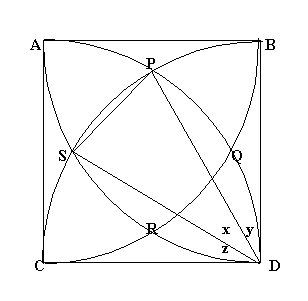#### You may also likeFour rods are hinged at their ends to form a convex quadrilateral. Investigate the different shapes that the quadrilateral can take. Be patient this problem may be slow to load.### Get Cross

A white cross is placed symmetrically in a red disc with the central square of side length sqrt 2 and the arms of the cross of length 1 unit. What is the area of the disc still showing?### Darts and Kites

Explore the geometry of these dart and kite shapes!

##### Age 14 to 16Challenge LevelThe solution below was done by Arun Iyer, age 17, S.I.A Junior College, South Indian Assoiate, Nr Station Dombivli (W), Thane Di 421 202, India and similar solutions were sent in by Tony Cardell and John Lesieutre, age 14, from State College Area High School, Pennsylvania, USA.

Given a square $ABCD$ of sides 10 cm, and using the corners as centres, construct four quadrants with radius 10 cm each inside the square. The arcs intersect at $P$, $Q$, $R$ and $S$ and we have to find the area $PQRS$ enclosed by the arcs.

Consider triangles $CPD$ and $SBD$. Both are equilateral triangles. ($CP = PD = CD$ = 10cm and $SB = SD = BD$ = 10cm). Therefore from the diagram $x+y = 60^\circ$ and $x+z = 60^\circ$. We know that $x+y+z= 90^\circ$ so from these three equations,

$x= 30^\circ =\pi /6$. The area of segment $PS$ = area of sector $PDS$ - area of triangle $PDS$. Call this area $\Delta$

$\Delta = {1 \over 2}(10)^2 (x - \sin x).$

By symmetry, there are 4 such equal segments. Hence, the total area of the segments is $4\Delta.$

Since the angles subtended by the arcs $PQ$, $QR$, $RS$ and $PS$ are equal, we get chords $L(PQ) = L(QR) = L(RS) = L(PR)$.

We can find $L(PS)$ by applying the cosine rule to triangle $PDS$, $L(PS)^2 = (10)^2 + (10)^2 - 2(10)^2 \cos x = 2(10)^2(1 - \cos x).$ $\mbox{Area of square PQRS} = L(PS)^2 = 2(10)^2(1 - \cos x).$

The required area of $PQRS$ = area of 4 segments + area of square $PQRS$.

\eqalign{ {\rm Area} &= 200(x - \sin x) + 200(1 - \cos x) \cr &= 200({\pi \over 6} - \sin{\pi \over 6} + 1 - \cos {\pi \over 6}) \cr &= 200({\pi \over 6} - {1\over 2}+ 1 - {\sqrt 3 \over 2})\cr &= 100(1 + {\pi \over 3} - \sqrt 3) \cr &= 31.54 \ {\rm cm^2 (rounded \ to \ 4 \ significant\ figures.)} }.